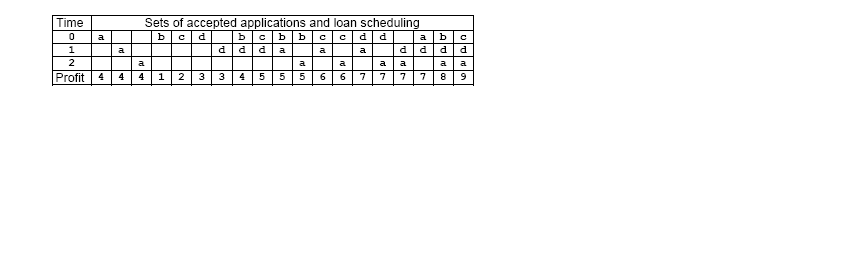# 往届 ACM 队训练题 (参考)

1030. Loan Scheduling

The North Pole Beach Bank has to decide upon a set App of mortgage applications. Each application a∈App has an acceptance deadline da, ie. the required loan must be paid at a time ta, 0≤ta≤da. If the application is accepted the Bank gets a profit pa. Time is measured in integral units starting from the conventional time origin 0, when the Bank decides upon all the App applications. Moreover, the Bank can pay a maximum number of L loans at any given time. The Bank policy if focussed solely on profit: it accepts a subset S ⊆ App of applications that maximizes the profit profit(S)=ΣPa(a∈S). The problem is to compute the maximum profit the Bank can get from the given set App of mortgage applications.

For example, consider that L=1, App={a,b,c,d}, (pa,da)=(4,2), (pb,db)=(1,0), (pc,dc)= (2,0), and (pd,dd)=(3,1). The table below shows all possible sets of accepted mortgage applications and the scheduling of the loan payments. The highest profit is 9 and corresponds to the set {c,d,a}. The loan requested by the application c is paid at time 0, the loan corresponding to d is paid at time 1, and, finally, the loan of a is paid at time 2.### 输入格式

Write a program that reads sets of data from an input text file. Each data set corresponds to a set of mortgage applications and starts with two integers: 0≤N≤10000 that shows the number of applications in the set, and 0≤L≤100 which shows the maximum number of loans the Bank can pay at any given time. Follow N pairs of integers pi di, i=1,N, that specify the profit 0≤pi0≤10000 and the deadline 0≤di0≤10000 of the application i. Input data are separated by white spaces, are correct, and terminate with an end of file.

### 输出格式

For each data set the program computes the maximum profit the Bank can get from the accepted mortgage applications corresponding to that data set. The result is printed on standard output from the beginning of a line. There must be no empty lines on output. An example of input/output is shown below.

### 样例

Input
4 1 4 2 1 0 2 0 3 1
7 2
200 1 200 1 100 0 1000 2 80 1
50 20 500 1
0 100
1 0 4 1000

Output
9
2050
0
0